# Approximation of heavy models using Radial Basis Functions

• Slides: 17Approximation of heavy models using Radial Basis Functions Graeme Alexander (Deloitte) Jeremy Levesley (Leicester) www. le. ac. ukThe problem • Calculate Value at Risk • Need to determine 0. 5 th percentile of insurer’s net assets in one year • Net assets = f(R 1, R 2, R 3, . . . Rn) • Many firms have previously calculated the percentiles of univariate distns, and aggregated using correlation matrix / copula approachMoving to Solvency II • For internal model approach, strongly encouraged to calculate the whole distribution of Net Assets, not just the percentile • It is a simple matter to generate 100, 000 simulations of (R 1, R 2, . . Rn) • However, evaluating f(r 1, r 2, . . rn) for a single realisation of the risk vector using the “heavy model” can take hours!! • Common approach: Run the heavy models on a small number of points, and interpolate to obtain estimator function f. E(r 1, r 2, . . , rn), known as a “lite model”Splines Linear spline approximation to sin(x) Combination of hat functions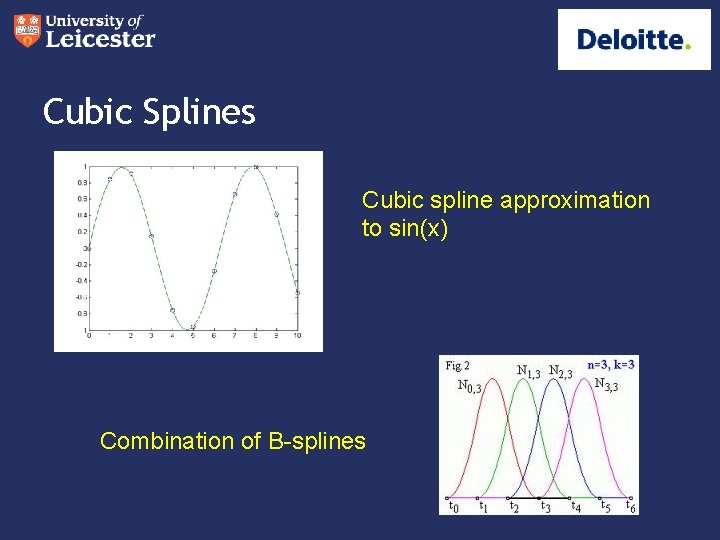Cubic Splines Cubic spline approximation to sin(x) Combination of B-splinesRadial basis function approximation • Set of points • A basis function • Approximation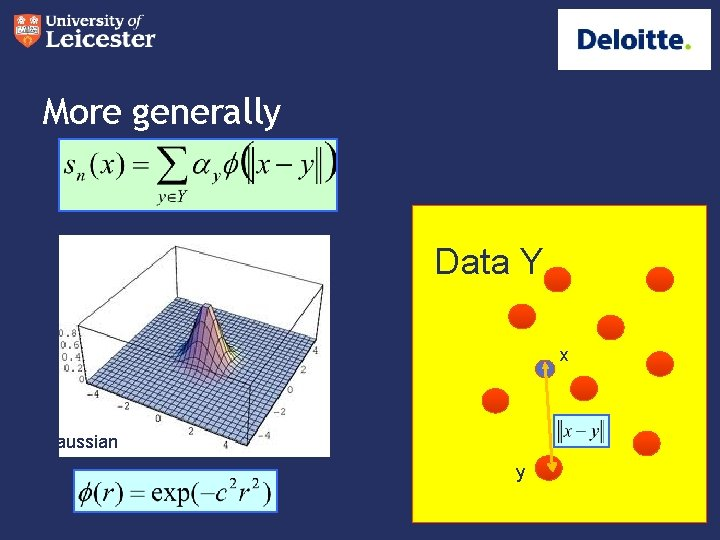More generally Data Y x Gaussian yHow to compute coefficients Interpolation Linear EquationsAn Example - annuity • Difficult to test our interpolation on real-life data due to the length of time it takes to run heavy models • So let’s take a simple product, a single life annuity, £ 1 payable p. a. • Assume just two risk factors, discount rate and mortality • Assume a constant rate of mortality 1/T in each future year. Thus, the cash flows are: (T-1)/T at the end of year 1, (T-2)/T at end of year 2, 1 / T at end of year T-1 • Allow T and disc to vary stochastically disc~ N (8%, 2. 5%2) T ~ N (20, 9)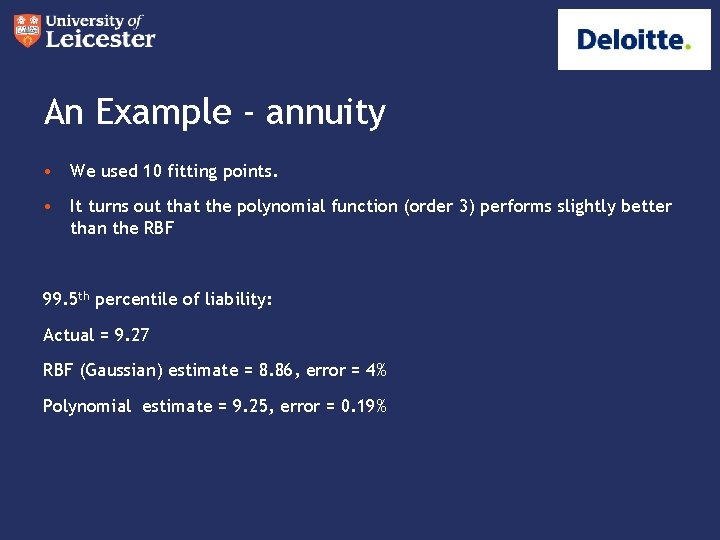An Example - annuity • We used 10 fitting points. • It turns out that the polynomial function (order 3) performs slightly better than the RBF 99. 5 th percentile of liability: Actual = 9. 27 RBF (Gaussian) estimate = 8. 86, error = 4% Polynomial estimate = 9. 25, error = 0. 19%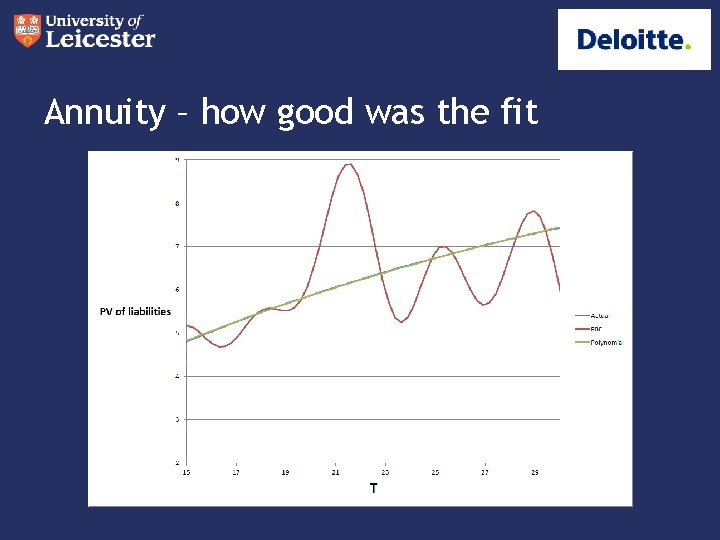Annuity – how good was the fit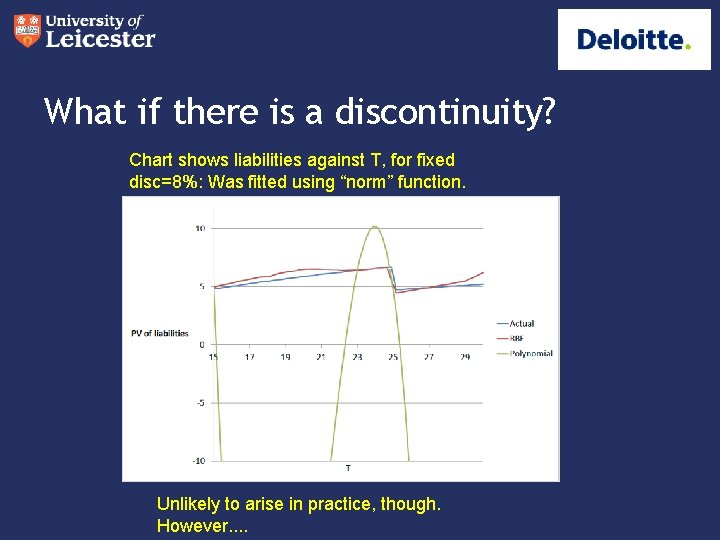What if there is a discontinuity? Chart shows liabilities against T, for fixed disc=8%: Was fitted using “norm” function. Unlikely to arise in practice, though. However. .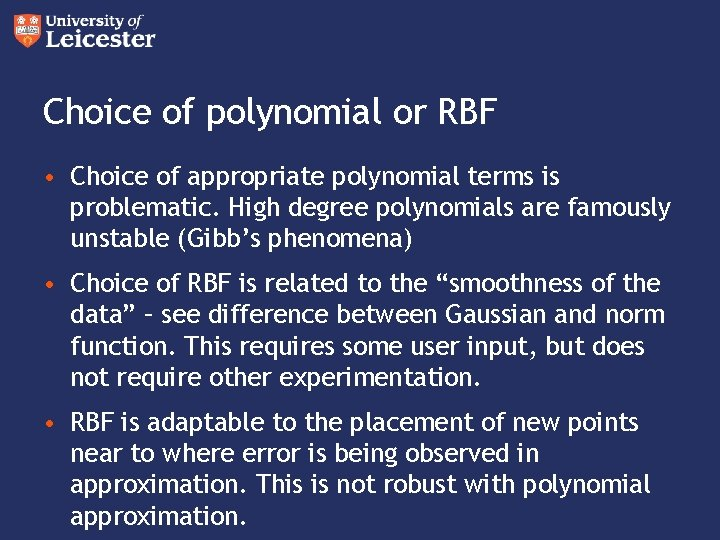Choice of polynomial or RBF • Choice of appropriate polynomial terms is problematic. High degree polynomials are famously unstable (Gibb’s phenomena) • Choice of RBF is related to the “smoothness of the data” – see difference between Gaussian and norm function. This requires some user input, but does not require other experimentation. • RBF is adaptable to the placement of new points near to where error is being observed in approximation. This is not robust with polynomial approximation.With profits • The realistic balance sheet includes a “cost of guarantees” • For example, suppose there is a guaranteed sum assured on the assets, equal to £ 500. • Crudely, we can model the cost of guarantees as a put option on the asset share. Assume that: v. Asset Share is £ 1, 000 v. Strike price (guarantee) is £ 500 v. Assets ~ N (1000, 3002), disc~ N (8%, 2. 5%2) This time the radial basis function (“norm”) does better: Actual = £ 83. 53 RBF estimate = £ 74. 6, error = 11% Polynomial estimate = £ 1, 735, error = 1978%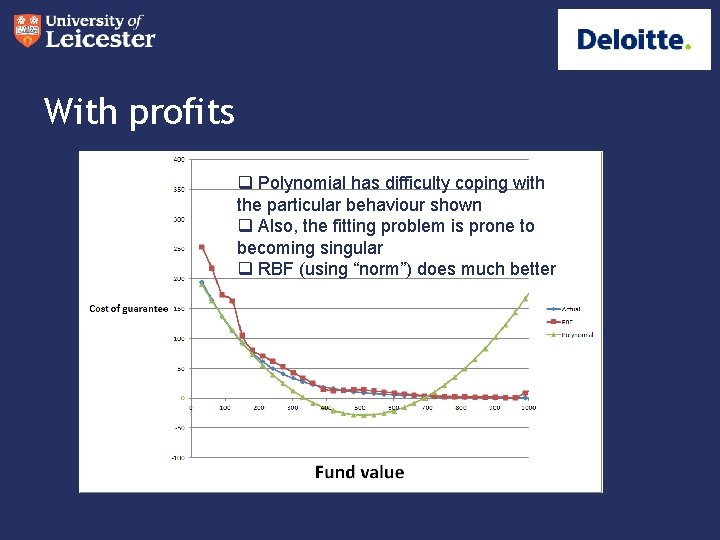With profits q Polynomial has difficulty coping with the particular behaviour shown q Also, the fitting problem is prone to becoming singular q RBF (using “norm”) does much better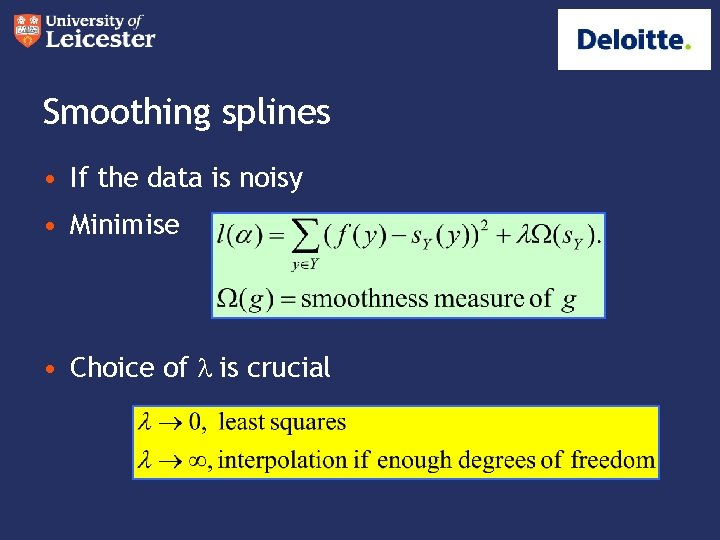Smoothing splines • If the data is noisy • Minimise • Choice of l is crucial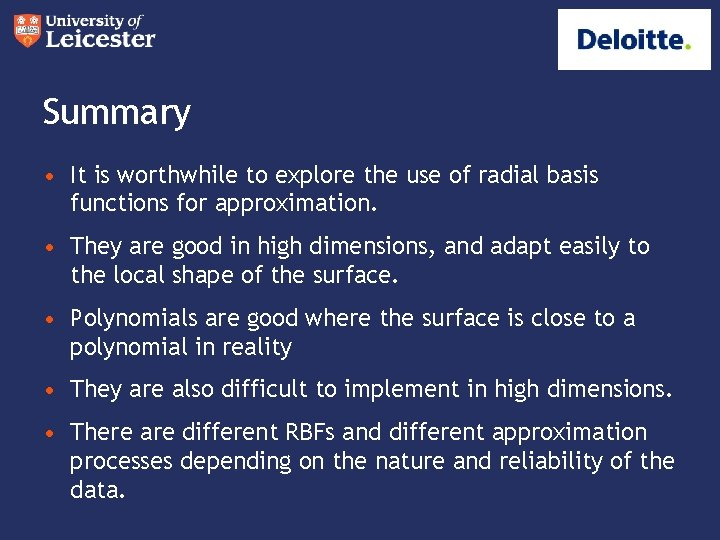Summary • It is worthwhile to explore the use of radial basis functions for approximation. • They are good in high dimensions, and adapt easily to the local shape of the surface. • Polynomials are good where the surface is close to a polynomial in reality • They are also difficult to implement in high dimensions. • There are different RBFs and different approximation processes depending on the nature and reliability of the data.